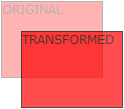# CSS 2D Transforms

## CSS 2D Transforms

CSS transforms allow you to move, rotate, scale, and skew elements.

Mouse over the element below to see a 2D transformation:

2D rotate

In this chapter you will learn about the following CSS property:

• `transform`

## Browser Support

The numbers in the table specify the first browser version that fully supports the property.

Property
transform 36.0
10.0
16.0
9.0
23.0

## CSS 2D Transforms Methods

With the CSS `transform` property you can use the following 2D transformation methods:

• `translate()`
• `rotate()`
• `scaleX()`
• `scaleY()`
• `scale()`
• `skewX()`
• `skewY()`
• `skew()`
• `matrix()`

Tip: You will learn about 3D transformations in the next chapter.

## The translate() MethodThe `translate()` method moves an element from its current position (according to the parameters given for the X-axis and the Y-axis).

The following example moves the <div> element 50 pixels to the right, and 100 pixels down from its current position:

### Example

div {
transform: translate(50px, 100px);
}
Try it Yourself »

## The rotate() MethodThe `rotate()` method rotates an element clockwise or counter-clockwise according to a given degree.

The following example rotates the <div> element clockwise with 20 degrees:

### Example

div {
transform: rotate(20deg);
}
Try it Yourself »

Using negative values will rotate the element counter-clockwise.

The following example rotates the <div> element counter-clockwise with 20 degrees:

### Example

div {
transform: rotate(-20deg);
}
Try it Yourself »

## The scale() MethodThe `scale()` method increases or decreases the size of an element (according to the parameters given for the width and height).

The following example increases the <div> element to be two times of its original width, and three times of its original height:

### Example

div {
transform: scale(2, 3);
}
Try it Yourself »

The following example decreases the <div> element to be half of its original width and height:

### Example

div {
transform: scale(0.5, 0.5);
}
Try it Yourself »

## The scaleX() Method

The `scaleX()` method increases or decreases the width of an element.

The following example increases the <div> element to be two times of its original width:

### Example

div {
transform: scaleX(2);
}
Try it Yourself »

The following example decreases the <div> element to be half of its original width:

### Example

div {
transform: scaleX(0.5);
}
Try it Yourself »

## The scaleY() Method

The `scaleY()` method increases or decreases the height of an element.

The following example increases the <div> element to be three times of its original height:

### Example

div {
transform: scaleY(3);
}
Try it Yourself »

The following example decreases the <div> element to be half of its original height:

### Example

div {
transform: scaleY(0.5);
}
Try it Yourself »

## The skewX() Method

The `skewX()` method skews an element along the X-axis by the given angle.

The following example skews the <div> element 20 degrees along the X-axis:

### Example

div {
transform: skewX(20deg);
}
Try it Yourself »

## The skewY() Method

The `skewY()` method skews an element along the Y-axis by the given angle.

The following example skews the <div> element 20 degrees along the Y-axis:

### Example

div {
transform: skewY(20deg);
}
Try it Yourself »

## The skew() Method

The `skew()` method skews an element along the X and Y-axis by the given angles.

The following example skews the <div> element 20 degrees along the X-axis, and 10 degrees along the Y-axis:

### Example

div {
transform: skew(20deg, 10deg);
}
Try it Yourself »

If the second parameter is not specified, it has a zero value. So, the following example skews the <div> element 20 degrees along the X-axis:

### Example

div {
transform: skew(20deg);
}
Try it Yourself »

## The matrix() MethodThe `matrix()` method combines all the 2D transform methods into one.

The matrix() method take six parameters, containing mathematic functions, which allows you to rotate, scale, move (translate), and skew elements.

The parameters are as follow: matrix(scaleX(), skewY(), skewX(), scaleY(), translateX(), translateY())

### Example

div {
transform: matrix(1, -0.3, 0, 1, 0, 0);
}
Try it Yourself »

## Exercise:

With the `transform` property, move the <div> element 100px to the right, and 200px down.

```<style>
div {
width: 100px;
height: 100px;
background-color: lightblue;
border: 1px solid black;
: ;
}
</style>

<body>
<div>This is a div</div>
</body>
```

Start the Exercise

## CSS Transform Properties

The following table lists all the 2D transform properties:

Property Description
transform Applies a 2D or 3D transformation to an element
transform-origin Allows you to change the position on transformed elements

## CSS 2D Transform Methods

Function Description
matrix(n,n,n,n,n,n) Defines a 2D transformation, using a matrix of six values
translate(x,y) Defines a 2D translation, moving the element along the X- and the Y-axis
translateX(n) Defines a 2D translation, moving the element along the X-axis
translateY(n) Defines a 2D translation, moving the element along the Y-axis
scale(x,y) Defines a 2D scale transformation, changing the elements width and height
scaleX(n) Defines a 2D scale transformation, changing the element's width
scaleY(n) Defines a 2D scale transformation, changing the element's height
rotate(angle) Defines a 2D rotation, the angle is specified in the parameter
skew(x-angle,y-angle) Defines a 2D skew transformation along the X- and the Y-axis
skewX(angle) Defines a 2D skew transformation along the X-axis
skewY(angle) Defines a 2D skew transformation along the Y-axis

W3Schools is optimized for learning and training. Examples might be simplified to improve reading and learning. Tutorials, references, and examples are constantly reviewed to avoid errors, but we cannot warrant full correctness of all content. While using W3Schools, you agree to have read and accepted our terms of use, cookie and privacy policy.